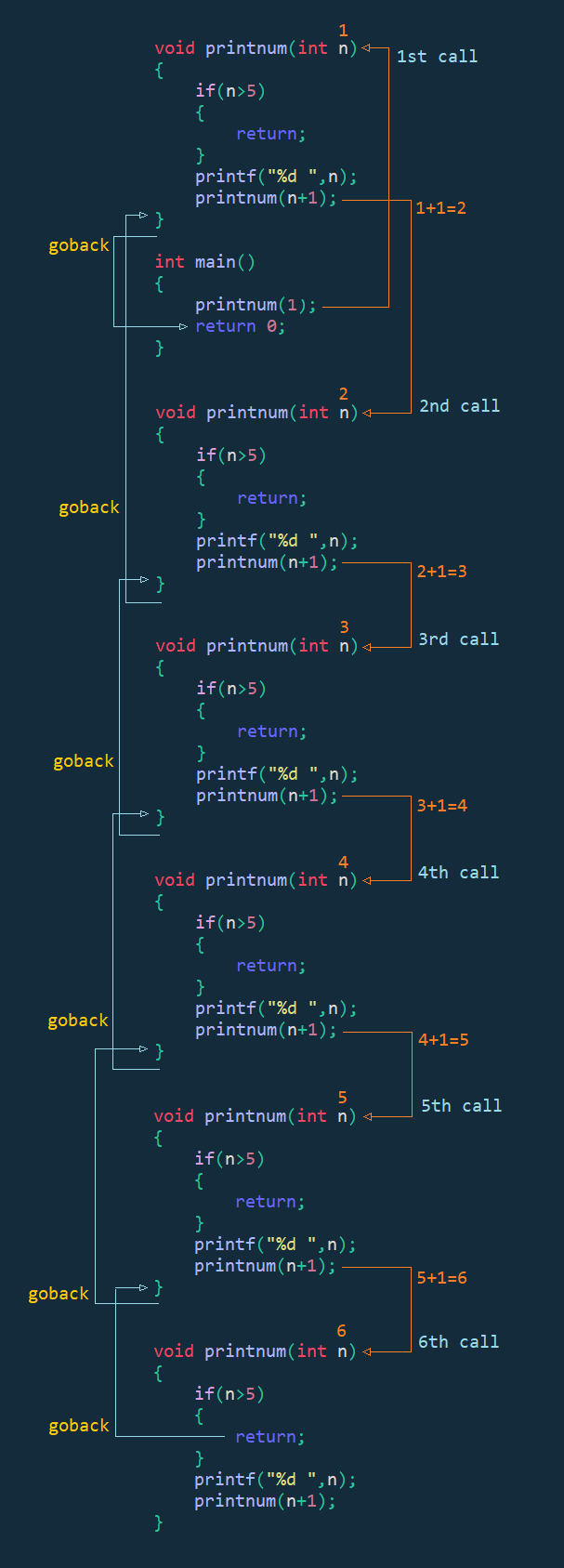# Recursive Function in C Programming

###### Function in C

In this lesson, we will understand what is Recursive Function in C Programming and how to create them along with some examples.

## What is Recursive Function in C

A Recursive Function in C is a function that calls itself repeatedly until the exit condition is satisfied.

Recursive Function is useful in solving complex problem easily. That's why it is used in data structure programs like binary tree, graph, etc.

Recursive Function is slower than loop because, during each recursive call, a new recursive function block is created in memory. Let's see how we can create a recursive function in C and how it works.## Recursive Function Creation

Below we have created a recursive function that prints the numbers from 1 to 5 using the recursive technique.

#### Example

``````#include <stdio.h>
#include <conio.h>

void printnum(int n)
{
if(n>5)
{
return;
}
printf("%d ",n);
printnum(n+1);
}

int main()
{
printnum(1);
return 0;
}``````

#### Output

`1 2 3 4 5`

In the above program, we have created a recursive function printnum() that accept a starting number and print all the numbers from the starting number up to 5 using the recursive technique.

## How Recursive Function Works

To understand how recursive function works. See the visual working of the above program given below.In the above image, we can see that we have called the function printnum(1) with a starting value 1 from the main() function.

In the 1st call, the function printnum() received the value 1 and check if it is greater than 5 or not. If not, then it prints the value on the screen and then called itself with the value 2.

In the 2nd call, the function printnum() received the value 2 and check if it is greater than 5 or not. If not, then it prints the value on the screen and then called itself with the value 3.

In the 3rd call, the function printnum() received the value 3 and check if it is greater than 5 or not. If not, then it prints the value on the screen and then called itself with the value 4.

In the 4th call, the function printnum() received the value 4 and check if it is greater than 5 or not. If not, then it prints the value on the screen and then called itself with the value 5.

In the 5th call, the function printnum() received the value 5 and check if it is greater than 5 or not. If not, then it prints the value on the screen and then called itself with the value 6.

In the 6th call, the function printnum() received the value 6 and check if it is greater than 5 or not. This time the value of n is greater than 5. So it returns back to the 5th block of the printnum() function.

From the 5th block, it returns back to the 4th block.

From the 4th block, it returns back to the 3rd block.

From the 3rd block, it returns back to the 2nd block.

From the 2nd block, it returns back to the 1st block.

From the 1st block, it returns back to the main() function and the program end.